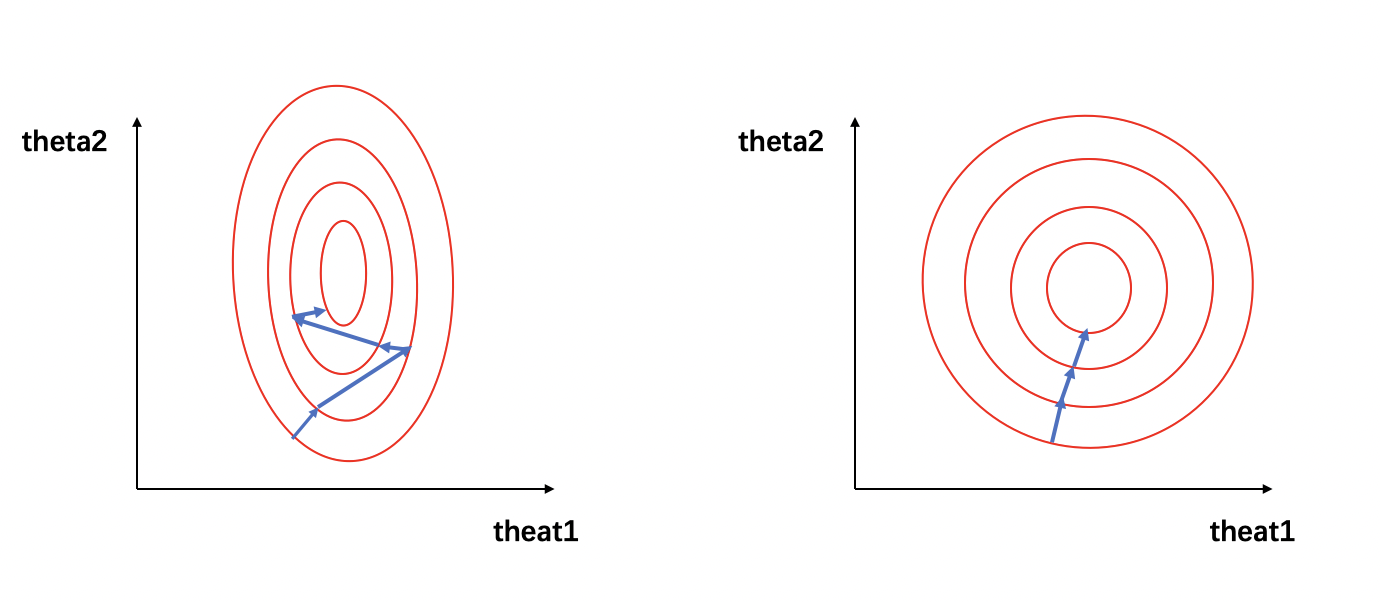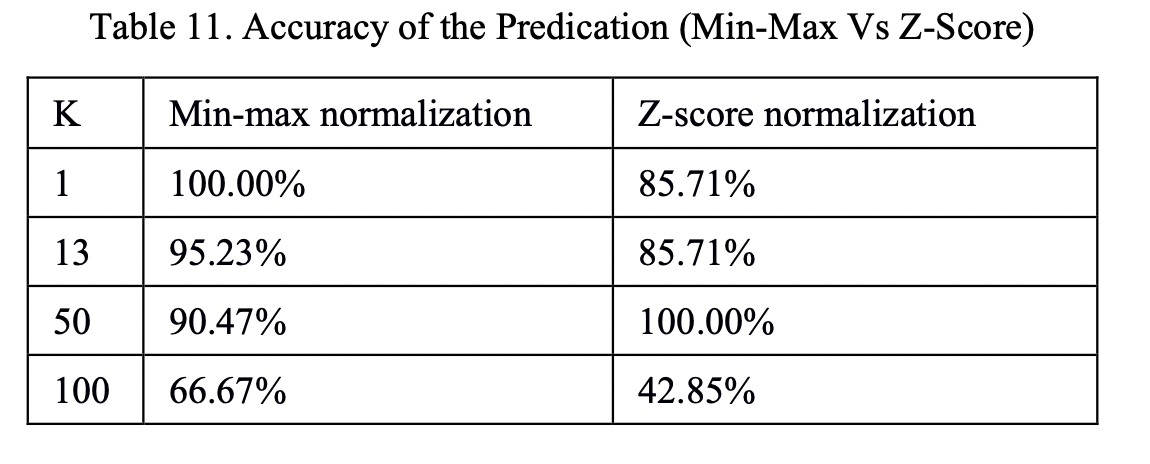## 2. 为什么要归一化

 - 归一化可以将有量纲转化为无量纲，同时将数据归一化至同一量级，解决数据间的可比性问题。在回归模型中，自变量的量纲不一致会导致回归系数无法解读或错误解读。在KNN、Kmeans等需要进行距离计算的算法中，量纲的量级不同可能会导致拥有较大量级的特征在进行距离计算时占主导地位，从而影响学习结果。

 - 数据归一化后，寻求最优解的过程会变得平缓，可以更快速的收敛到最优解。详见3.为什么归一化能提高求解最优解的速度

## 3. 为什么归一化能提高求解最优解的速度

\begin{align} y &= \theta_1x_1 + \theta_2x_2 \\ J &= (\theta_{1}x_{1} + \theta_{2}x_{2} - y_{label})^2 \end{align}

$$J = (1000\theta_{1}+3\theta_{2} - y_{label})^2$$$$J(x) = (0.2\theta_{1} + 0.3\theta_{2} - y_{label})^2$$

## 4. 归一化的类型

 - Min-max normalization （Rescaling）：

$$x^{'} = \frac{x - min(x)}{max(x) - min(x)}$$

 - Mean normalization：

$$x^{'} = \frac{x - mean(x)}{max(x) - min(x)}$$

 - Z-score normalization （Standardization）：

$$x^{'} = \frac{x - \mu}{\sigma}$$

 - 非线性归一化

• 对数归一化：

$$x^{'} = \frac{\lg x}{\lg max(x)}$$

• 反正切函数归一化：

$$x^{'} = \arctan(x) * \frac{2}{\pi}$$

• 小数定标标准化（Demical Point Normalization）:

$$x^{'} = \frac{x}{10^j}$$

## 5. 不同归一化的使用条件

 - Min-max归一化和mean归一化适合在最大最小值明确不变的情况下使用，比如图像处理时，灰度值限定在 [0, 255] 的范围内，就可以用min-max归一化将其处理到[0, 1]之间。在最大最小值不明确时，每当有新数据加入，都可能会改变最大或最小值，导致归一化结果不稳定，后续使用效果也不稳定。同时，数据需要相对稳定，如果有过大或过小的异常值存在，min-max归一化和mean归一化的效果也不会很好。如果对处理后的数据范围有严格要求，也应使用min-max归一化或mean归一化。

 - Z-score归一化也可称为标准化，经过处理的数据呈均值为0，标准差为1的分布。在数据存在异常值、最大最小值不固定的情况下，可以使用标准化。标准化会改变数据的状态分布，但不会改变分布的种类。特别地，神经网络中经常会使用到z-score归一化。

 - 非线性归一化通常被用在数据分化程度较大的场景，有时需要通过一些数学函数对原始值进行映射，如对数、反正切等。## 6. 归一化和标准化的联系与区别

$$x^{'} = \frac{x - b}{a} = \frac{x}{a} - \frac{b}{a} = \frac{x}{a} - c$$

 - 归一化不会改变数据的状态分布，但标准化会改变数据的状态分布；

 - 归一化会将数据限定在一个具体的范围内，如 [0, 1]，但标准化不会，标准化只会将数据处理为均值为0，标准差为1。

## References

 - Comparative Analysis of KNN Algorithm using Various Normalization Techniques；Amit Pandey，Achin Jain.

Last modification：September 18th, 2021 at 10:28 am